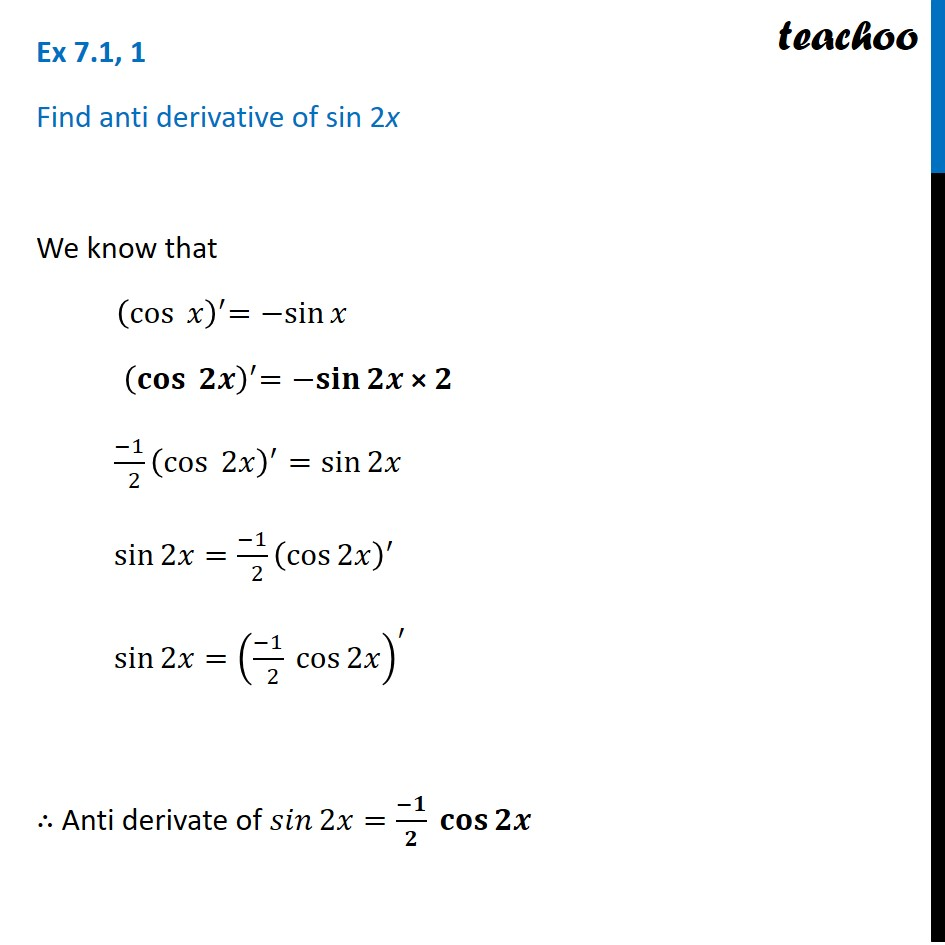Using Trignometric Formulaes

Chapter 7 Class 12 Integrals
Concept wiseLearn in your speed, with individual attention - Teachoo Maths 1-on-1 Class

### Transcript

Ex 7.1, 1 Find anti derivative of sin 2x We know that 〖 (cos⁡〖 𝑥〗 )〗^′=〖−sin〗⁡𝑥 〖 (𝐜𝐨𝐬⁡〖 𝟐𝒙〗 )〗^′=〖−𝐬𝐢𝐧〗⁡𝟐𝒙 × 𝟐 (−1)/( 2) (cos⁡〖 2𝑥〗 )^′=sin⁡2𝑥 sin⁡2𝑥=(−1)/( 2) (cos⁡2𝑥 )^′ sin⁡2𝑥=((−1)/( 2) cos⁡2𝑥 )^′ ∴ Anti derivate of 𝑠𝑖𝑛⁡2𝑥=(−𝟏)/𝟐 𝐜𝐨𝐬⁡𝟐𝒙